Test: Long And Short - 2

# Test: Long And Short - 2 - Class 4

Test Description

## 20 Questions MCQ Test Mathematics for Class 4: NCERT - Test: Long And Short - 2

Test: Long And Short - 2 for Class 4 2023 is part of Mathematics for Class 4: NCERT preparation. The Test: Long And Short - 2 questions and answers have been prepared according to the Class 4 exam syllabus.The Test: Long And Short - 2 MCQs are made for Class 4 2023 Exam. Find important definitions, questions, notes, meanings, examples, exercises, MCQs and online tests for Test: Long And Short - 2 below.
Solutions of Test: Long And Short - 2 questions in English are available as part of our Mathematics for Class 4: NCERT for Class 4 & Test: Long And Short - 2 solutions in Hindi for Mathematics for Class 4: NCERT course. Download more important topics, notes, lectures and mock test series for Class 4 Exam by signing up for free. Attempt Test: Long And Short - 2 | 20 questions in 40 minutes | Mock test for Class 4 preparation | Free important questions MCQ to study Mathematics for Class 4: NCERT for Class 4 Exam | Download free PDF with solutions
 1 Crore+ students have signed up on EduRev. Have you?
Test: Long And Short - 2 - Question 1

### The measuring tape shows that the length of the plane is 8. What is the unit of this length?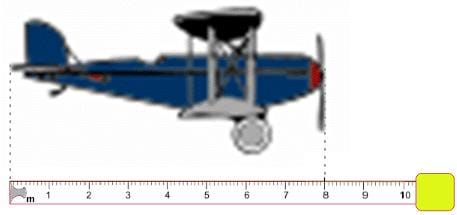Detailed Solution for Test: Long And Short - 2 - Question 1

The unit of measurement is m.
So the plane is 8 m long.

Test: Long And Short - 2 - Question 2

### The ruler shows that the length of the pencil is 12. What is the unit of this length?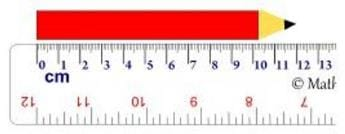Detailed Solution for Test: Long And Short - 2 - Question 2

The unit of measurement is cm.
So the pencil is 12 cm long.

Test: Long And Short - 2 - Question 3

### Imagine you are given the following four measuring instruments: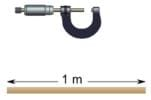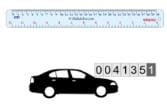A. A micrometer, which is a special instrument used by engineers to accurately measure to the nearest millimeter or even more precisely. B. A 30 cm ruler, which is the ruler you usually use in school. C. A stick exactly one meter long. D. An odometer, which is an instrument used in a car to measure distances in kilometers. Which instrument would you use to roughly measure the length of your classroom?

Detailed Solution for Test: Long And Short - 2 - Question 3

You would not be looking for an accurate answer, so you would use a stick exactly one meter long, and see how many times you need to use the stick.

Test: Long And Short - 2 - Question 4

Two traffic signs mark the bridge in my village: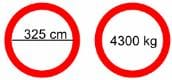These signs indicate the maximum vehicle width and the maximum vehicle weight allowed on the bridge.

Which one of the following trucks is allowed to cross that bridge?

Detailed Solution for Test: Long And Short - 2 - Question 4

'Maximum' means the greatest that is allowed.
In case A, the weight (4400 kg) is too much.
In case B, the weight (4400 kg) is too much.
In case C, the width (330 cm) is too much.
In case D, the width (325 cm) is on the maximum and the weight (4250 kg) is below the maximum. So D is allowed to cross the bridge.

Test: Long And Short - 2 - Question 5

Imagine you are given the following four measuring instruments: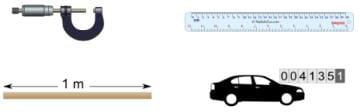A. A micrometer, which is a special instrument used by engineers to accurately measure to the nearest millimeter or even more precisely.
B. A 30 cm ruler, which is the ruler you usually use in school.
C. A stick exactly one meter long.
D. An odometer, which is an instrument used in a car to measure distances in kilometers.

Which instrument would a telephone engineer use to measure the size of a small component?

Detailed Solution for Test: Long And Short - 2 - Question 5

He would have to measure accurately, so he would use a micrometer.

Test: Long And Short - 2 - Question 6

This pencil has length 120. What is the unit of this length?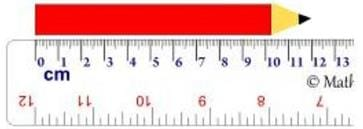Detailed Solution for Test: Long And Short - 2 - Question 6

The pencil is 12 cm long.
12 cm = 120 mm
So, the unit of measurement is mm.

Test: Long And Short - 2 - Question 7

Imagine you are given the following four measuring instruments: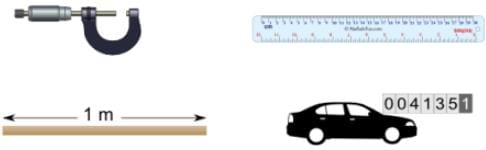A. A micrometer, which is a special instrument used by engineers to accurately measure to the nearest millimeter or even more precisely.
B. A 30 cm ruler, which is the ruler you usually use in school.
C. A stick exactly one meter long.
D. An odometer, which is an instrument used in a car to measure distances in kilometers.

Which instrument would a taxi driver in a large city use to measure the distance from the city center to the airport?

Detailed Solution for Test: Long And Short - 2 - Question 7

He would  measure the distance using the taxi's odometer.

Test: Long And Short - 2 - Question 8

The measuring tape shows that the length of the plane is 800. What is the unit of this length?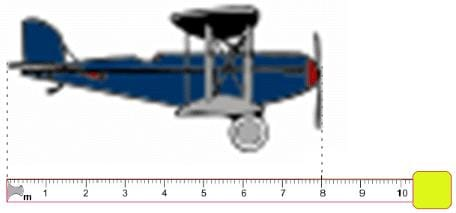Detailed Solution for Test: Long And Short - 2 - Question 8

The plane is 8 m long.
8 m = 800 cm
So the unit of measurement is cm.

Test: Long And Short - 2 - Question 9

Imagine you are given the following four measuring instruments:A. A micrometer, which is a special instrument used by engineers to accurately measure to the nearest millimeter or even more precisely.
B. A 30 cm ruler, which is the ruler you usually use in school.
C. A stick exactly one meter long.
D. An odometer, which is an instrument used in a car to measure distances in kilometers.

Which instrument would you use to measure the length of your index finger?

Detailed Solution for Test: Long And Short - 2 - Question 9

You would use an ordinary ruler to measure the length of your index finger to the nearest millimeter.

Test: Long And Short - 2 - Question 10

Which unit would you most likely use to measure the width of the cover of a paperback book?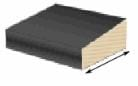Detailed Solution for Test: Long And Short - 2 - Question 10

The width of the cover of a paperback book is usually about 11 centimeters, so you would use centimeters.

Test: Long And Short - 2 - Question 11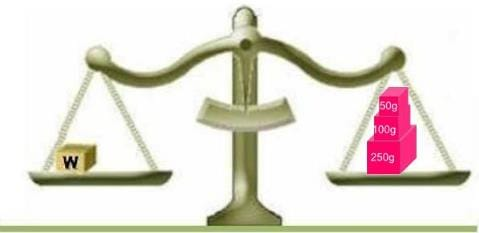The scales are balanced.
What is the value of the weight W in grams?

Detailed Solution for Test: Long And Short - 2 - Question 11

W = 50g + 100 g + 250 g = 400 g

Test: Long And Short - 2 - Question 12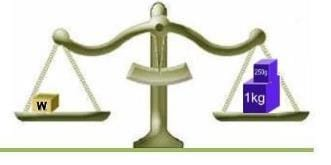The scales are balanced.
What is the value of the weight W in grams?

Detailed Solution for Test: Long And Short - 2 - Question 12

W = 1 kg + 250 g = 1000 g + 250 g = 1250 g

Test: Long And Short - 2 - Question 13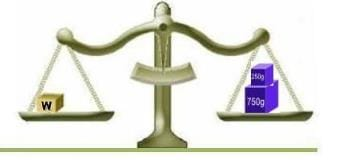The scales are balanced.
What is the value of the weight W in grams?

Detailed Solution for Test: Long And Short - 2 - Question 13

W = 250g + 750 g = 1000 g

Test: Long And Short - 2 - Question 14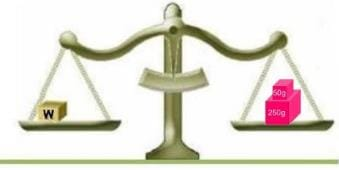The scales are balanced.
What is the value of the weight W in grams?

Detailed Solution for Test: Long And Short - 2 - Question 14

W = 50g + 250 g = 300 g

Test: Long And Short - 2 - Question 15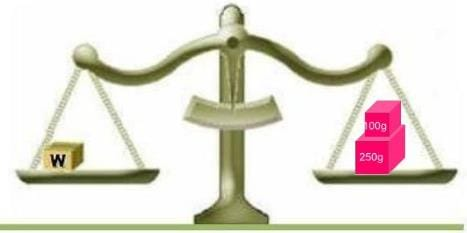The scales are balanced.
What is the value of the weight W in grams?

Detailed Solution for Test: Long And Short - 2 - Question 15

W = 100g + 250 g = 350 g

Test: Long And Short - 2 - Question 16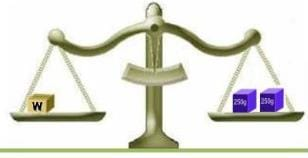The scales are balanced.
What is value of the weight W in grams?

Detailed Solution for Test: Long And Short - 2 - Question 16

W = 250g + 250 g = 500 g

Test: Long And Short - 2 - Question 17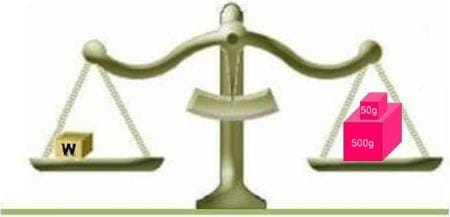The scales are balanced.
What is the value of the weight W in grams?

Detailed Solution for Test: Long And Short - 2 - Question 17

W = 50g + 500 g = 550 g

Test: Long And Short - 2 - Question 18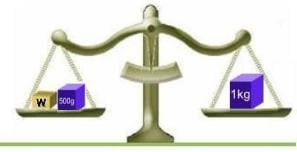The scales are balanced.
What is value of the weight W in grams?

Detailed Solution for Test: Long And Short - 2 - Question 18

W and 500 g together balance with 1 kg = 1000 g.
So W must be 1000g − 500 g = 500g.

Test: Long And Short - 2 - Question 19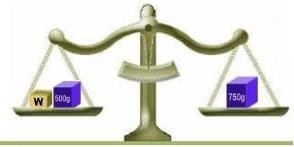The scales are balanced.
What is value of the weight W in grams?

Detailed Solution for Test: Long And Short - 2 - Question 19

W and 500 g together balance with 750g
So W must be 750g − 500 g = 250g.

Test: Long And Short - 2 - Question 20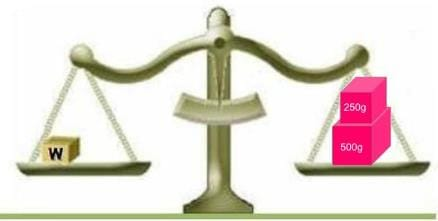The scales are balanced.
What is the value of the weight W in grams?

Detailed Solution for Test: Long And Short - 2 - Question 20

W = 250g + 500 g = 750 g

## Mathematics for Class 4: NCERT

25 videos|84 docs|41 tests
Information about Test: Long And Short - 2 Page
In this test you can find the Exam questions for Test: Long And Short - 2 solved & explained in the simplest way possible. Besides giving Questions and answers for Test: Long And Short - 2, EduRev gives you an ample number of Online tests for practice

## Mathematics for Class 4: NCERT

25 videos|84 docs|41 tests